## Kirchoff's current law

Algebraic sum of electric currents flowing toward to a point or a branching of a circuit, equal to the current that out of the point.

Written by equation

Σ I = 0

See electric current branching scheme as in the circuit belowCurrents toward to the point A marked as positive (+), the points are IA, IB and ID.
Currents out of the point A marked as negative (-), that is IC
The equation becomes:
IA + IB - IC + ID = 0
IA + IB + ID = IC

Example question:
In the circuit above, it is known that IA = 10 A, IB = 6 A, IC = 18 A. What ID current is given to the circuit?

completion:
IA + IB - IC + ID = 0
10 + 6-18 + ID = 0
ID = 18 - 16 = 2 A

## Series connection of capacitors

The effective capacitance or total value (Ct) a connection two or more capacitors in series, is calculated using the equation ever wrote for parallel connection to the resistance, use the formula:

1/Ct = 1/C1 + 1/C2 + 1/C3 + · · · + 1/Cn

where:
C1, C2, C3 = capacitance of each component
Cn = capacitance of the n components

Example question:
See the picture below, how many effective capacitance is given to circuit?completion:
1/Ct = 1/C1 + 1/C2 + 1/C3
1/Ct = 1 / 47 + 1 / 22 + 1 / 10
1/Ct = 1724/10340
1724Ct = 10 340
Ct = 10340/1742
Ct = 6 μF

conclusion:
1. Equation series connection of capacitors equal to parallel connection of resistance
2. Effective capacitance or total value (Ct) a series capacitor circuit is always smaller than the smallest value of capacitor in the circuit.

## Parallel connection of capacitors

Connecting two or more capacitors in parallel can be equated by summing the plate area of each capacitor. For this reason, the effective capacitance or total value (Ct) a parallel circuit of capacitor is equal to the sum capacitance of all capacitors in the circuit. Equation parallel connection of capacitors is equal to ever wrote for series connection to the resistance, can use the formula:

Ct = C1 + C2 + C3 + · · · + Cn

where:
C1, C2, C3 = capacitance of each component
Cn = capacitance of the n components

Example question:In the picture above, how many effective capacitance is given to circuit?

completion:
Ct = C1 + C2 + C3
Ct = 47 μF + 10 μF + 2.2 μF = 59.2 μF

Conclusion:

1. Equation parallel connection of capacitors equal to series connection of resistance
2. Effective capacitance or total value (Ct) a parallel capacitor circuit is always greater than the largest value of capacitor in the circuit.

## How to read, or calculating capacitor value?

Capacitance value is often printed on the capacitor body, because there is not enough space on bodies of small-sized capacitors, the capacitance values ​​must be encoded.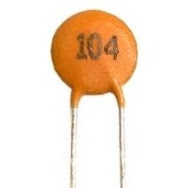Code that is used consists of three digits. The first two digits of code of is the first two digits of the value of the capacitor is concerned, in units pikofarad (pF). The third digit represents the number of zeros contained in the back of two digits earlier.

Example question 1:
What is the value of capacitance of capacitors shown in the picture above?

completion:
The capacitor has a code 104, meaning that 10 followed by 4 pieces of zeros behind it. By using the value conversion units, then
C = 100000 pF
C = 100 nF
C = 0.1 μF
Capacitor values ​​obtained in the image above is 0.1 μF

Example question 2:
How much the value of the capacitor with code 223, 122 and 471?

completion:
1. The code 223 means that 22 followed by 3 pieces of zeros behind it. This value are 22 000 pF, equal to 22 nF
2. The code 122 means that 12 followed by 2 pieces of zeros behind it. This value is 1200 pF, equal to 1.2 nF
3. The code 471 means that 47 is followed by 1 piece of zero behind it. This value are 470 pF, equal to 0.47 nF

## Capacitor and capacitance of capacitor

A capacitor consists of two sides of a metal plate with an insulating layer (partition) located between these two plates. Insulator layer that is used can be a thin plastic plate, but in some types of capacitors, this layer is air.Picture above shows examples of the capacitor and picture below is a symbol of the capacitor.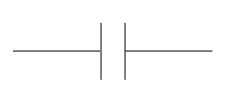If a capacitor is connected to a DC source, the electrons will gather at the plate that is connected to the negative terminal of the source. These electrons will reject electrons that exist on the opposite plate. Electrons are rejected will flow towards the positive terminal of the source.A capacitor is connected like this to the source, will be charged immediately. Voltages between the two plates is equal to the voltage power source. When the capacitor is released from the source, the capacitor retains the charge. Due to insulator layer on the capacitor, the current can not flow through the capacitor. Capacitor will remain charged up to infinite time. For this reason, capacitors are very useful for keep electrical charge.Capacitance
The ability of a capacitor to keep electrical charge is called capacitance of capacitor, with the symbol C. Unit for capacitance is farad, which is symbolized by F. One farad is defined as the amount of electrical charge that can be saved (in units of coulombs) by unit of voltage.

Capacitance of capacitor = electrical charge / voltage

Example:
Electrical charge keep in a capacitor is 6 coulombs. Voltages between the two plates is 2 V. What is the value of capacitance?

Completion:
Capacitance of capacitor = 6 / 2 = 3 F

Capacitor values
Capacitors made ​​with a value similar to the reference values ​​for the resistor. Capacitors have a higher tolerance than the resistor, so that we do not find it necessary to create as much as 24 pieces of value within that range. Capacitance values ​​in this range are:
1,0     1,2     1,5     1,8     2,2     2,7     3,3     3,9     4,7     5,6     6,8     8,2
These values ​​are repeated again with a multiple of 10.

## How to measuring voltage and current electricity?

By having AVO meter, multimeter, or multi tester, we can measure the voltage and amperage. However, it is necessary to note the measurement procedure on that tool.

Measurement procedure
1. Before you connect the meter to the circuit or the load to be measured, consider whether using a source of AC or DC?
2. Set the selector knob on the range you want
3. If meter has auto ranging capability, you only need to select the amount of electricity (current or voltage)
4. If you have any doubt in determining the proper range, choose the highest range
5. Connect the meter to the circuit, or just attach both probes at two points in the circuit, note the polarity (+ and -), black to negative, and red to positive
6. Read the value of measurement is shown (note that you avoid parallax errors in multi tester analog) and record
7. If meter shows the reading of a very small value, you can reduce the range of measurements until you get results that can be read, but first disconnect meter from the circuit
8. Remove the meter from the circuit after you get a correct value readings
9. Rotate the selector knob to the off position or turn it off

Measuring Voltage and Current Electricity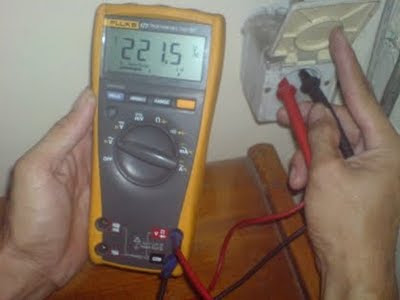Measuring voltage, multimeter is connected in parallel with the voltage source circuit. Meanwhile measuring the electric current, multimeter must be connected in series with the circuit. See the picture below## Voltage divider circuit

Voltage divider circuit is also known as potential divider circuit. Input to a voltage divider circuit is Vin.

Vin voltage will drive current (I) through both resistors. Because of both resistors connected in series, then the same current will flows pass through each resistor.Effective resistance of two series resistors are R1 + R2. Drop voltage across this combination of both resistor is a Vin, according to Ohm's Law, the current flowing is
I = Vin / (R1 + R2)
Voltage at R2 becomes
Vout = I x R2
Substituting the current (I) with the first equation
Vout = Vin x R2 / (R1 + R2)

This equation is an equation to calculate the output voltage generated by a voltage divider circuit. By selecting two resistor with resistance values suitable, we can get the value of any output voltage within the range 0 to Vin (Volt).

## Repair USB optical mouse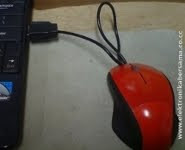Less than half a year has a netbook, optical mouse has been damaged. Damage that occurs, the red indicator light is on but the mouse cursor was quiet, the pointer does not move following the mouse movements.

I did some trouble shooting, and I found that the USB cable connector at the edge was problem.

In the cable mouse consists of four small-sized wires, which is two 5 VDC power supply cable (plus and minus) and two data cables. Because of frequent moves, one of the existing data cable is broken. To fix this, prepare the tools:

• AVO meter or Multi Tester
• The knife cutter
• Solder and tin
• Isolation

Following steps:

1. Cut the cable at least 10 cm from the edge of the USB socket
2. Use AVO meter or multi tester to mark the color and position of the cable (identification), as shown below3. Open the USB connector cover, with a vertical cut using a cutter knife, and try cut in the middle so that the result looks neat
4. Remove the solder cable 10 cm that had been cut at the USB socket terminal
5. Solder mouse cable to the USB socket terminal. Note the color and position of cable, must be precise not to reverse
6. Isolate the cover. Examples of the results as shown below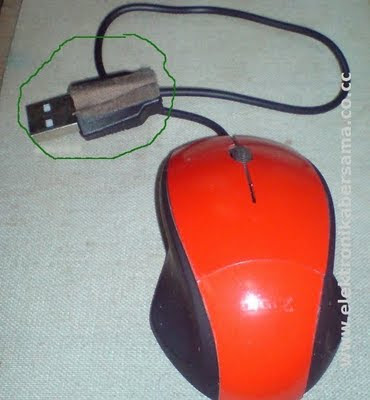Reference: Elektronikabersama

## How to calculate mixture circuit (series and parallel) on the resistors?

In a the circuit mixes, some of the resistors are connected in series while the other is connected in parallel. To calculate the value of Rt in series-parallel circuit, the first method is to find the groups of resistors are all connected in series or all of which are connected in parallel.

Draw back the circuit, by replacing each group of resistors into an equivalent resistor. Gradually, simplify the circuit to produce an Rt, or the resistance of a single end.

Example question:
What is the value of Rt, or the equivalent resistance of the circuit resistor mixture below?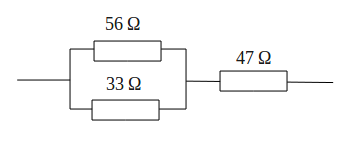Completion:
On the image above the resistance value of 56 Ω and 33 Ω are connected in parallel, then parallel connection is connected in series with 47 Ω.

Create Rt1 equivalent resistance for parallel connection resistance 56 Ω and 33 Ω, with a parallel formula
1/Rt = 1/R1 + 1/R2 + 1/R3 + · · · + 1/Rn, then
1/Rt1 = 1 / 56 + 1 / 33
1/Rt1 = 89/1848
89Rt1 = 1848
Rt1 = 1848/89
Rt1 = 20.8 Ω

Parallel connection of resistance 56 Ω and 33 Ω can be replaced with an equivalent resistance of 20.8 Ω. This simplification produces two resistance are connected in series, which is 20.8 Ω and 47 Ω.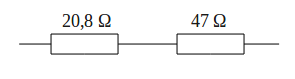Equivalent series resistance value according to the series formula
Rt = R1 + R2 + R3 + · · · + Rn, then
Rt = 20.8 + 47
Rt = 68.7 Ω

Thus obtained Rt or an equivalent resistance for 67.8 Ω## Code printed on the resistors

Code printed on the resistors are used to replace the resistor color code. This kind of code also known as code BS1852.

The code printed is using one of the three letters below, to indicate the unit and decimal point position of the resistance value. These three letters are:
R = Ohm
K = kilo Ohm
M = Mega Ohm

See the following tableThere is also a letter that is added at the end of the code to indicate the values ​​of tolerance, which is
G = ± 2%
J = ± 5%
K = ± 10%
L = ± 20%

Example question:
What is the resistance value of resistors are marked with code
(a) 33K, (b) 4M7, (c) 2k2, (d) 1R8J, (e) 27KK

Completion:
(a) 33 kΩ
(b) 4.7 MΩ
(c) 2.2 kΩ
(d) 1.8 Ω ± 5%
(e) 27 kΩ ± 10%